Warning: include(highlight.inc.php): failed to open stream: No such file or directory in /usr/share/nginx/html/drucken.php on line 12

Warning: include(): Failed opening 'highlight.inc.php' for inclusion (include_path='.:/usr/local/lib/php') in /usr/share/nginx/html/drucken.php on line 12

## LED DIMMING WITH PWM### PWM for power control

A DC (Direct Current) device is powered by a constant voltage and normally its power consumption depends on the voltage level applied. As example an electric heater can be considered as a resistor with resistance R. If U is the supply voltage, the power consumption is

 P = U * I = U  * (U)/ R = (U2/ R

There are many electric devices that approximately behave like (variable) resistors, among them DC motors, filament lamps and LEDs. If such a device is powered by a digital output of a microcontroller system, the supply voltage level is either 0 or VCC. The voltage of a digital output port is typically VCC = 3.3V or 5V, but the device supply voltage can easily be decreased by a series resistor or increased by a simple transistor or IC driver circuit.

But it is not so easy to control the power (speed of the motor, luminosity of the bulb or LED) in a purely digital system, due to the lack of analog output ports. The trick is the following: For the overall power consumption only the mean value of the supply voltage is of importance. If within a given short period T of time, say T = 50 ms, the voltage is applied only for a fraction ton of the period and turned of for the rest of the period toff = T - ton, the (mean) power is controlled by selecting the duty cycle D = ton/T (often given in percent from 0% to 100%).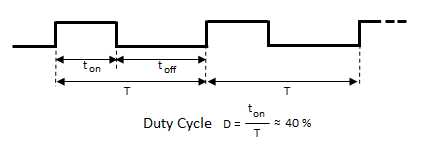It is rather easy to generate a PWM signal by software. You even don't have to write your own Python code, because a PWM generator is included in the GPIO library. Sometimes it is a too heavy burden for the processor to generate the PWM signal, then you better use a special designed external PWM chip like the PCA9685 (consult the datasheet here).

### Experiment 1: Dimming of a LED

 Aim: Attach a LED (do not forget the serial resistor) to the Raspberry Pi and create a little light show by periodically increasing the luminosity and dropping it to zero using PWM. Realize some other schedule of light variation. Program:[►] ```# PWM1.py # LED dimming import RPi.GPIO as GPIO import time P_LED = 22 # adapt to your wiring fPWM = 50 # Hz (not higher with software PWM) def setup(): global pwm GPIO.setmode(GPIO.BOARD) GPIO.setup(P_LED, GPIO.OUT) pwm = GPIO.PWM(P_LED, fPWM) pwm.start(0) print "starting" setup() duty = 0 isIncreasing = True while True: pwm.ChangeDutyCycle(duty) print "D =", duty, "%" if isIncreasing: duty += 10 else: duty -= 10 if duty == 100: isIncreasing = False if duty == 0: isIncreasing = True time.sleep(1) ``` Highlight program code (Ctrl+C copy, Ctrl+V paste) Remarks: To use a GPIO port as PWM output, you first define it as output and then create a PWM object pwm with given PWM period T. The PWM frequency fPWM is the number of periods (cycles) per second (1 / T) and it should be high enough to avoid flickering. The maximum value is limited to about 50 Hz because of the software based generation of the signal. To set the duty cycle, you invoke the function (method) ChangeDutyCycle(duty) of the pwm object.

### Experiment 2: Selecting the color using a 3-color LED

By adding three color components R (red), G (green) and B (blue), any RGB color can be emitted (like right now on your color screen, have a look with a magnifying glass). A color LED consists of three small red, green and blue emitting LEDs mounted into a single housing. The LEDs have a common anode A (positive) or cathode C (negative) and 3 leads called R, G, B.

Common devices:

 Luminex SSL-LX5097 (Datasheet) SMD 5050 RGB (KY-009 module) KY--016 module with serial resistors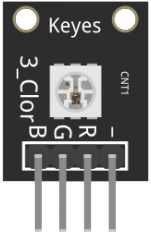Aim:
Use a 3-color LED you have at hand and create a color light show by displaying random RGB colors every 200 ms.

Circuitry: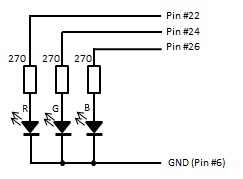Program:[►]

```# PWM2.py
# Set RGB color

import RPi.GPIO as GPIO
import time
import random

P_GREEN = 24   # ditto
P_BLUE = 26    # ditto
fPWM = 50      # Hz (not higher with software PWM)

def setup():
global pwmR, pwmG, pwmB
GPIO.setmode(GPIO.BOARD)
GPIO.setup(P_RED, GPIO.OUT)
GPIO.setup(P_GREEN, GPIO.OUT)
GPIO.setup(P_BLUE, GPIO.OUT)
pwmR = GPIO.PWM(P_RED, fPWM)
pwmG = GPIO.PWM(P_GREEN, fPWM)
pwmB = GPIO.PWM(P_BLUE, fPWM)
pwmR.start(0)
pwmG.start(0)
pwmB.start(0)

def setColor(r, g, b):
pwmR.ChangeDutyCycle(int(r / 255 * 100))
pwmG.ChangeDutyCycle(int(g / 255 * 100))
pwmB.ChangeDutyCycle(int(b / 255 * 100))

print "starting"
setup()
while True:
r = random.randint(0, 255)
g = random.randint(0, 255)
b = random.randint(0, 255)
print r, g, b
setColor(r, g, b)
time.sleep(0.2)
```
Highlight program code (Ctrl+C copy, Ctrl+V paste)

Remarks:

The serial resistors must be chosen to limit the current to about 10 mA (depending on the maximum allowed LED current, but not more than 16 mA, because of the current limitation of the GPIO ports).

### Experiment 3: RGB LED strips with MOSFET driver

RGB LED strips are composed by an array of color LEDs (mostly type SMD 5050). Normally 3 LEDs of the same color and a current limiting resistors are in series and driven with 12V DC. Several such elements are wired in parallel and the total current depends on the length of the strip, but may easily reach 1 A for each color input lead. This is far more than a GPIO output can deliver. Therefore some kind of buffer or driver device is needed that handles the 12V/1A supply. Commonly the driver is built with bipolar transistors or MOSFETs. Pairs of transistors can also be arranged in a IC package called Darlington array.

Common GPIO drivers:

 Bipolar Transistor MOSFET Darlington Array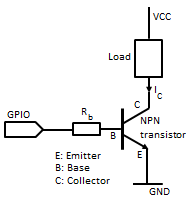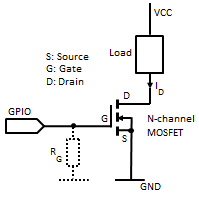Example: Transistor: 2N2222A Rb = 1 kOhm VCC (max): 40 V IC (max): 0.8 A Example: MOSFET: FQP30N06L RG = 100 kOhm (optional to set gate to GND when open) VCC (max): 60 V ID (max): 60 A (with heat sink) Example: IC: ULN2003A (Pin #9 connection optional for inductive loads) VCC(max): 50 V IL(max): 0.5 A (for each of 7 channels)

Aim:
Buy a cheap RGB LED strip and a 12 V DC (stabilized) power supply from the next mall. Use 3 MOSFET drivers (e.g. FQP30N06L) and realize the circuit shown below (solder or use a breadboard). Write a funny program for a colorful light show.

Circuitry:As first approach you may use the same program as above, but it's up to you to create a much better performance.

### Experiment 4: Fully addressable RGB LED strip

 (to be done)